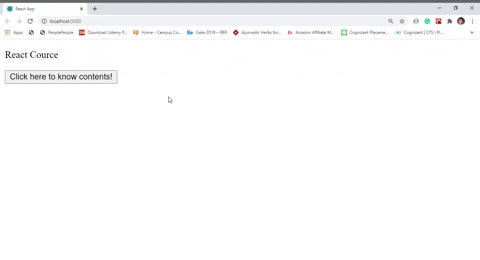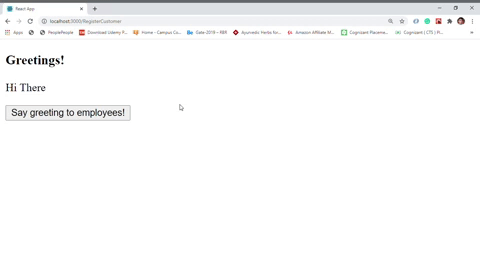Open In App
Related Articles

# How to avoid binding by using arrow functions in callbacks in ReactJS?

In React class-based components when we use event handler callbacks, it is very important to give special attention to the ‘this’ keyword. In these cases the context this is undefined when the callback function actually gets invoked that’s why we have to bind the context of this. Now if binding all the methods of each class is very annoying. Instead of binding we can use the inline arrow function since the arrow function does not have its own value of this, it uses the parent or public value. Using the inline arrow function we can get rid of annoying method binding every time and also the code looks very packed and organized.

Example 1: This example illustrates how to use arrow functions in callbacks

index.js:

•

## Javascript

 `import React from ``'react'``import ReactDOM from ``'react-dom'``import App from ``'./App'` `ReactDOM.render(, document.querySelector(``'#root'``))`

•

App.js :

•

## Javascript

 `import React, { Component } from ``'react'` `class App extends Component {``  ``// Default props``  ``static defaultProps = {``    ``courseContent : [``      ``'JSX'``, ``'React Props'``, ``'React State'``, ``'React Lifecycle Methods'``,``      ``'React Event Handlers'``, ``'React Router'``, ``'React Hooks'``, ``'Readux'``,``      ``'React Context'``    ``]``  ``}``  ` `  ``constructor(props){``    ``super``(props)``    ` `    ``// Set initial state``    ``this``.state = {msg : ``'React Course'``, content:``''``}``  ``}` `  ``// Return an unordered list of contents``  ``renderContent(){``    ``return` `(``      ``
``        ``{``/* map over all the contents and``            ``return some JSX for each  */``}``        ``{``this``.props.courseContent.map(content => (``          ``
• {content}
• ``        ``))}``      ``
``    ``)``  ``}``  ` `  ``render(){``    ``const button = !``this``.state.content &&``    `` {``        ``// Update state``        ``this``.setState({``          ``msg : ``'Course Content'``,``          ``content : ``this``.renderContent()``        ``})``      ``}}``    ``>``      ``Click here to know contents!``    ````    ``return` `(``      ``
``        `  `

{``this``.state.msg}

`  `        `  `

{``this``.state.content}

`  `        ``{button}``      ``
``    ``)``  ``}``}` `export ``default` `App`

•

Output :Example 2 :

index.js :

•

## Javascript

 `import React from ``'react'``import ReactDOM from ``'react-dom'``import App from ``'./App'` `ReactDOM.render(, document.querySelector(``'#root'``))`

•

App.js :

•

## Javascript

 `import React, { Component } from ``'react'` `class App extends Component{``  ``// Default props``  ``static defaultProps = {``    ``name : [``'John'``, ``'Alex'``, ``'Bob'``]``  ``}``  ``constructor(props){``    ``super``(props)``    ``// Nnitialize count state``    ``this``.state = {msg : ``'Hi There'``, count:0}``  ``}` `  ``render(){``    ``return``(``      ``
``        ``

Greetings!

``        `  `

{``this``.state.msg}

`  `        `` {``            ``this``.setState(st => (``              ``st.msg = `\${st.msg}, \${``this``.props.name[st.count]}`,``              ``st.count += 1``            ``))``          ``}}``        ``>``          ``Say greeting to employees!``        ````      ``
``    ``) ``  ``}``}``export ``default` `App`

•

Output :